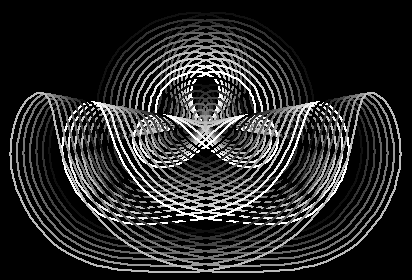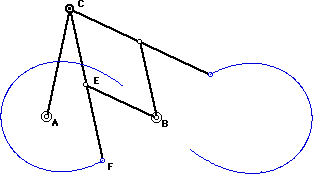# Lemniscate of BernoulliConchoids of Lemniscate of Bernoulli, colored with varing levels of gray. Conchoids of Lemniscate

## History

Studied by Jacob Bernoulli .

## Description

Lemniscate of Bernoulli is a special case of Cassinian oval. That is, the locus of points P, such that distance[P,F1] * distance[P,F2] == (distance[F1,F2]/2)^2, where F1, F2 are fixed points called foci. It is analogous to the definition of ellipse, where sum of two distances is replace by product.

## Formula

• Parametric: Cos[t]/(1+Sin[t]^2) {1, Sin[t]}, 0 < t ≤ 2 π.
• Polar: r^2 == Cos[2 * θ].
• Cartesian: (x^2 + y^2)^2 == (x^2-y^2).

Foci are at {-1/Sqrt,0}, {1/Sqrt,0}

## Properties

### Lemniscate as a Cissoid

Lemniscate of Bernoulli can be generated as a cissoid of two circles.

Step by step description:

1. Let there be a circle with radius r.
2. Let there be a point O, r*Sqrt distant from the center of the circle.
3. Draw a line passing through O and the circle. Let the intersections be Q1, Q2.
4. Let there be a vector with origin at O whose length is distance[Q1,Q2].
5. The locus of the vector is one loop of the lemniscate of Bernoulli. The other loop is symmetric with respect to O.

### Relation to Rectangular Hyperbola

Its inversion and negative pedal with respect to its center is the rectangular hyperbola. It is also the envelope of circles with centers on a rectangular hyperbola and each circle passing the hyperbola's center.

### Slicing a Torus

Lemniscate of Bernoulli is the intersection of a plane tangent to the inner ring of a torus whose inner radius equals to its radius of generating circle. (See: Cassinian oval).

### Construction of Tangent and Normal

The normal of any point P on the curve makes a angle 2 theta with the radius vector and 3 theta with the polar axis. The tangent of inclination is 2 theta + π/2.

Lemniscate of Bernoulli can be generated by these linkages. On the left: AB == ND == OD == c, AO == AN == BD == c/Sqrt. P and Q are midpoints of line OD and ND respectively. The point P traces half a lemniscate and half a cicle. Same with Q. On the right: AB == AC == a, CE == BE == EF == a/SqrtLemniscate Linkage 1 Lemniscate Linkage 2

### Trivia

The math symbol for infinity is shaped like a lemniscate. It was first used by John Wallis in 1655 in his De Sectionibus conicis (See: A history of mathematical notations By Florian Cajori. Buy at amazon)

## Related Web Sites

Robert Yates: Curves and Their Properties .

The MacTutor History of Mathematics archive